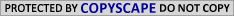# Stock Market Terms Letter VStock Market Terms Letter V

Value Line Arithmetic Composite Average (\$VLE): The Value Line Arithmetic Composite Average[\$VLE] is an equally weighted price index of all stocks in the Value Line Investment Survey. It is referred to as "arithmetic" because the index is computed by finding the mean or simple average.

Value Line Geometric Composite Average (\$XVG): The Value Line Geometric Composite Average[\$XVG] is an equally weighted price index of all stocks in the Value Line Investment Survey. It is referred to as "geometric" because the index is computed by finding the geometric average. A geometric average is calculated by taking the nth root of the product of n terms.

Visual Analysis: A form of analysis that utilizes charts and market indicators to determine market direction.

Volatility: A measurement of change in price over a given period. It is usually expressed as a percentage and computed as the annualized standard deviation of the percentage change in daily price. The more volatile a stock or market, the more money an investor can gain (or lose!) in a short time.

Volatility (Implied): A key variable in most option pricing models, including the famous Black-Scholes Option Pricing Model. Other variables usually include: security price, strike price, risk-free rate of return and days to expiration. If all other variables are equal, the security with the highest volatility will have the highest option prices. Many Nasdaq[\$COMPQ] and tech stocks have higher volatilities than NYSE[\$NYA] and non-tech stocks, and their options are also priced accordingly. One method of measuring volatility is by finding the standard deviation of the underlying security. However, the standard deviation cannot always explain the volatility that is implied by an option's price. Many times the price of an option will reflect more volatility than that measured by the standard deviation. This led to the notion of implied volatility, which is based on option prices. If the option price is known, then plugging in all variables and solving for volatility will yield the implied volatility.

Volatility Index (\$VIX): The Market Volatility Index measures the volatility of the market. A recent news story described it as "the options market's gauge of investor fear." Traders use VIX as a general inverse indicator of market volatility and sentiment. High numbers mean that there's excess bearishness, and low numbers indicate excess bullishness. The VIX is updated intraday by the Chicago Board Options Exchange (CBOE), using Standard & Poors 500 Index (SPX) bid/ask quotes. It was created in 1993.

Volume: The number of trades in a security over a period of time. On a chart, volume is usually represented as a histogram (vertical bars) below the price chart. The NYSE and Nasdaq measure volume differently. For every buyer, there is a seller: 100 shares bought = 100 shares sold. The NYSE would count this as one trade and as 100 shares of volume. However, the Nasdaq would count each side of the trade and as 200 shares volume.

Volume Oscillator: See Percentage Volume Oscillator.

_______________________________________________________________

Recommended Stock Trading Resources and Tools

_______________________________________________________________

 Trading Master Plan E-book:Learn to Trade the Stock Market!Discover How to Make Great Profits Part-Time Four FREE Bonuses IncludedInstant Download.Market Wizards:One of the Most Fascinating Books Ever Written About Wall Street!Understand What it Takes to Become a Successful Stock Trader